Next: Optimal grouping of a Up: The problems Previous: The problems

## Minimal triangulation of a convex polygon

A convex polygon has interior angles that are each strictly less than 180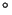(or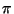radians, if you like). A triangulation of a convex polygon is formed by drawing diagonals between non-adjacent vertices (corners), provided you never intersect another diagonal (except at a vertex), until all possible choices of diagonals have been used (see Figure 1).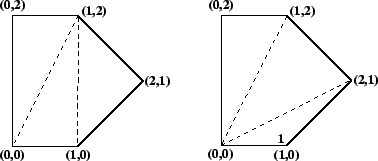Suppose a convex polygon has vertices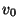,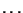,. In any triangulation we can assign a weight to each triangle: the length of its perimeter.2Let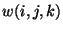denote the length of the perimeter of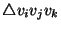. The cost of a triangulation is just the sum of the weights of its component triangles. We want to find a triangulation with the minimum cost.

Here's a sketch of how to find a minimum cost triangulation of a convex polygon with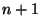vertices:

1. Number the vertices of your polygon from 0 to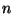.

2. Denote the cost of a minimal triangulation (which you haven't yet found) of your polygon as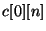. Similarly, your polygon contains sub-polygons with vertices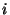through(ifis at least 2 greater than), and you can denote the weight of a minimal triangulation of such a sub-polygon as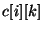.

3. Possible values offall into two cases. Case one: if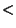then the polygon with vertices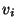has fewer than 3 vertices, and no triangulation is possible, so an appropriate minimum triangulation cost is 0. Case two: if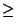, then there are one or more choices of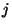where. For each choice of, calculate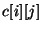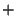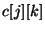-- the minimum over all appropriateis. These two cases give the following recursive formula: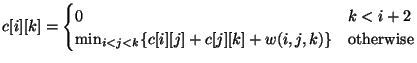(1)

Record which indexcorresponds to the minimum (you might denote it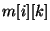).

4. Suppose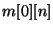is denoted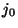, then you know that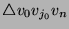is part of a minimal triangulation. You can find more triangles in this minimal triangulation using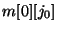and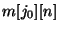. Continue until you've found all triangles in a minimal triangulation.

Although it is possible to build up a table of valuesiteratively (as described in your course notes), for this assignment you must findrecursively, using memoization (mentioned in lecture) to prevent redundant calculations of the same value.Next: Optimal grouping of a Up: The problems Previous: The problems
Danny Heap 2002-11-27